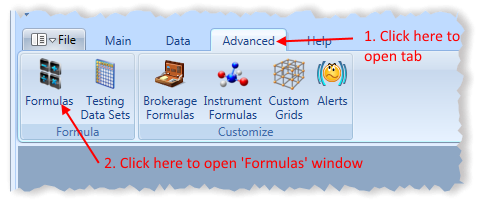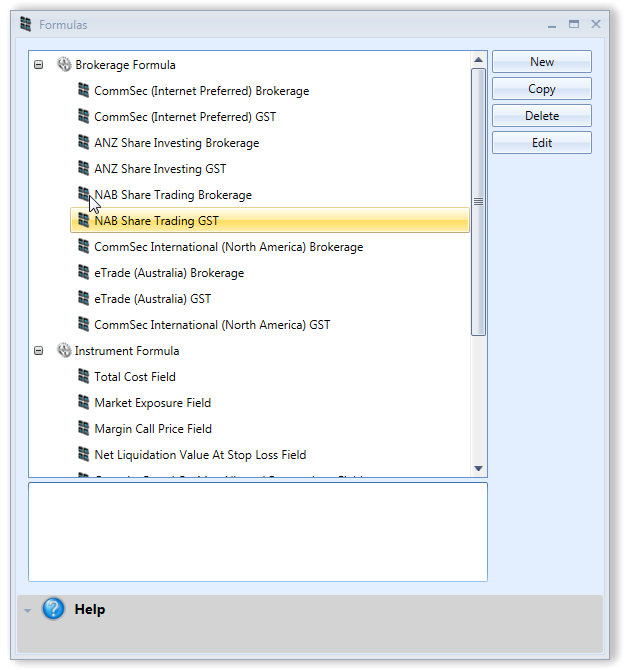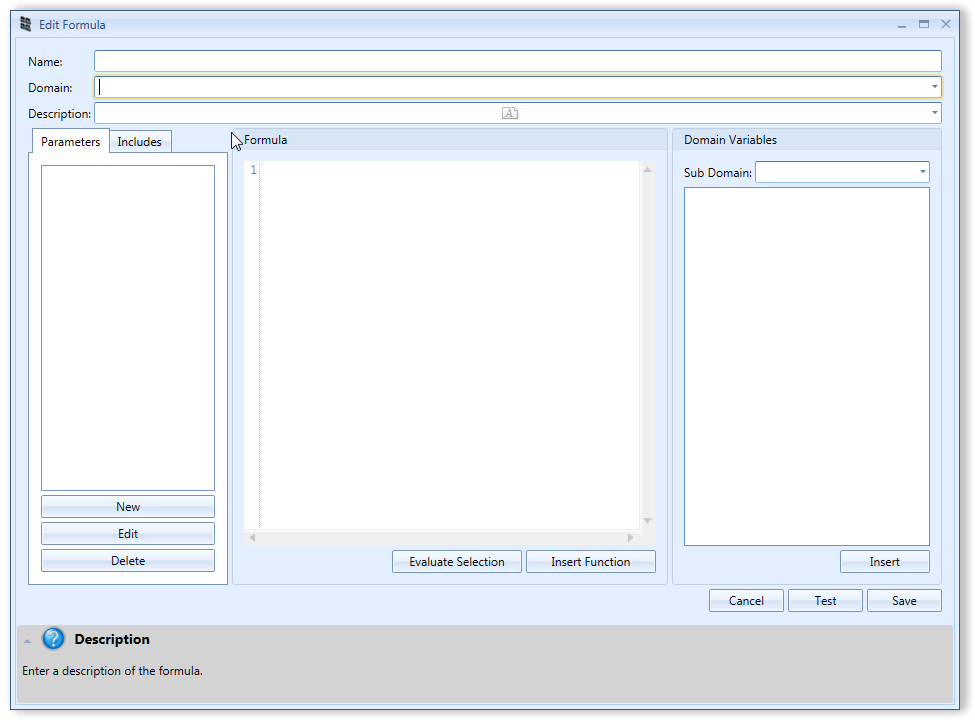# The Formula Language

The programming language used in Stock Portfolio Organizer is based on JavaScript (ECMA 5) with a decimal type added. In fact you can use any JavaScript (ECMA 5) code in your formulas and it should work with no changes required. For that reason instead of writing yet another JavaScript tutorial we will assume you are familiar with the JavaScript programming language. If not, you can search for one in Google.

The default number type in JavaScript and most other languages is unsuitable for financial calculations as it can introduce subtle rounding errors. For example, if you run the formula 1.345 + 1.123 the result will be 2.4679999352 instead of the expected result 2.468. A decimal number is specified by the ‘M’ suffix. So the previous formula could have been written as 1.345M + 1.123M which would yield the correct answer since it uses the decimal type.

# Creating a Formula

To create a formula to be used in a particular domain click the ‘Formulas’ toolbar item which can be found in the ‘Advanced’ tab in the main window.This should open the following window.Next click on ‘New’, which should open the following window.Name – Formula name.

Domain – The place where this formula can be used and the variables that can be accessed.

Description – Formula description.

Sub Domain – Some domains have different variables depending on the context. For example, the ‘Instrument Formula’ domain has sub domains for Forex, Futures and Share Margin. Each root domain has a variable that allows you to distinguish what sub domain the formula is running in. In the ‘Instrument Formula’ domain the ‘InstrumentType’ can be used to determine what domain a formula is running in.

Parameters – User configurable variables. For example, if you would like the user to be able to specify the stop loss amount to your formula you can create a decimal parameter named ‘StopLoss’. For example, if the formula was then used in a Custom Grid, the user can change the parameter by selecting the Custom Grid and clicking ‘Setup Parameters’ in the ‘Custom Grids’ window.

Includes – Include some code in your formula. Sometimes you may want to include a large library of code to use in your formula without cluttering the main formula box. You can do this by creating an include.

Evaluate Selection – Allows you to evaluate some code you have selected. This can be helpful for debugging purposes.